### 前言

var code = “47f56ed3-1701-4c13-a755-19dd0f5d101e”

### 一、const

#### 1.什么是const

const是C语言中的一种修饰符，和宏定义一样，只要定义了该变量就不能再改变了。

#### 2.const的使用

const type variate = value;

• type：类型
• variate：变量名
• value：值
const 修改后的变量的值不能对它在进行赋值了，例如下面的代码
#include <stdio.h>
int main(){
const int a = 5;
a = 6;
return 0;
}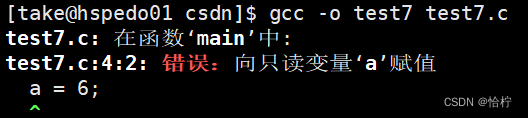### 二、const修饰一级指针

const 放在变量中是很简单的，如果将 const 放在指针中的情况会很复杂，现在来一一讲解。

#### 1.const放在 * 左边

#include <stdio.h>
int main(){
int a = 5, b = 10;
const int* pa = &a;
return 0;
}


#include <stdio.h>
int main(){
int a = 10, b = 20;
const int* pa = &a;
pa = &b;
printf("%d\n", *pa);
return 0;
}#include <stdio.h>
int main(){
int a = 10, b = 20;
const int* pa = &a;
*pa = b;
printf("%d\n", *pa);
return 0;
}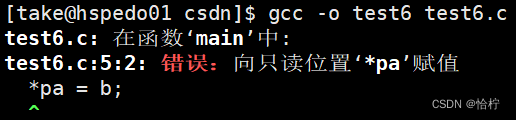#### 2.const在*右边

#include <stdio.h>
int main(){
int a = 5, b = 10;
int* const pa = &a;
return 0;
}


#include <stdio.h>
int main(){
int a = 5, b = 10;
int* const pa = &a;
pa = &b;
return 0;
}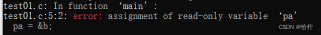### 三、const修饰二级指针

#### 1.const修饰**前

#include <stdio.h>
int main(){
int a = 5, b = 10;
int* pa = &a;
int* pb = &b;
const int ** ppa = &pa;
**ppa = 28;
return 1;
}


const 修饰在 ** 前修饰的内容是 **ppa ，也就是 **ppa 中所对应的内容不会被修改，所以第7行语句会报一个错误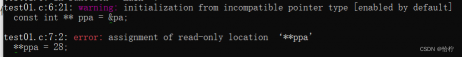#include <stdio.h>
int main(){
int a = 5, b = 10;
const int* pa = &a;
int* pb = &b;
const int ** ppa = &pa;
**ppa = 28;
return 1;
}


#### 2.const修饰**中间

#include <stdio.h>
int main(){
int a = 5, b = 10;
int* pa = &a;
int* pb = &b;
int * const * ppa = &pa;
*ppa = &b;
return 1;
}


const 修饰在 ** 中间，本质上修饰 *ppa 中的内容，如果改变 *ppa 中存放的地址，会报错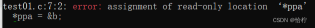#### 3.const修饰**右边

#include <stdio.h>
int main(){
int a = 5, b = 10;
int* pa = &a;
int* pb = &b;
int** const ppa = &pa;
ppa = &pb;
return 1;
}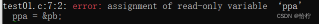const 对于指针的修饰情况有很多，级数越高的指针，产生的全款就会越多，所以一般都只考虑 const 到二级指针的情况，更高级的指针不会考虑。